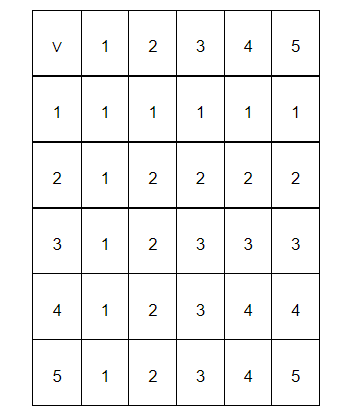# Consider the binary operation ∨ on the set {1, 2, 3, 4, 5} defined by a ∨b = min {a, b}.

Question:

Consider the binary operation $\vee$ on the set $\{1,2,3,4,5\}$ defined by $a \vee b=\min \{a, b\}$. Write the operation table of the operation $v$.

Solution:

The binary operation ∨ on the set {1, 2, 3, 4, 5} is defined as  b = min {ab}

&mnForE; ab ∈ {1, 2, 3, 4, 5}.

Thus, the operation table for the given operation ∨ can be given as: